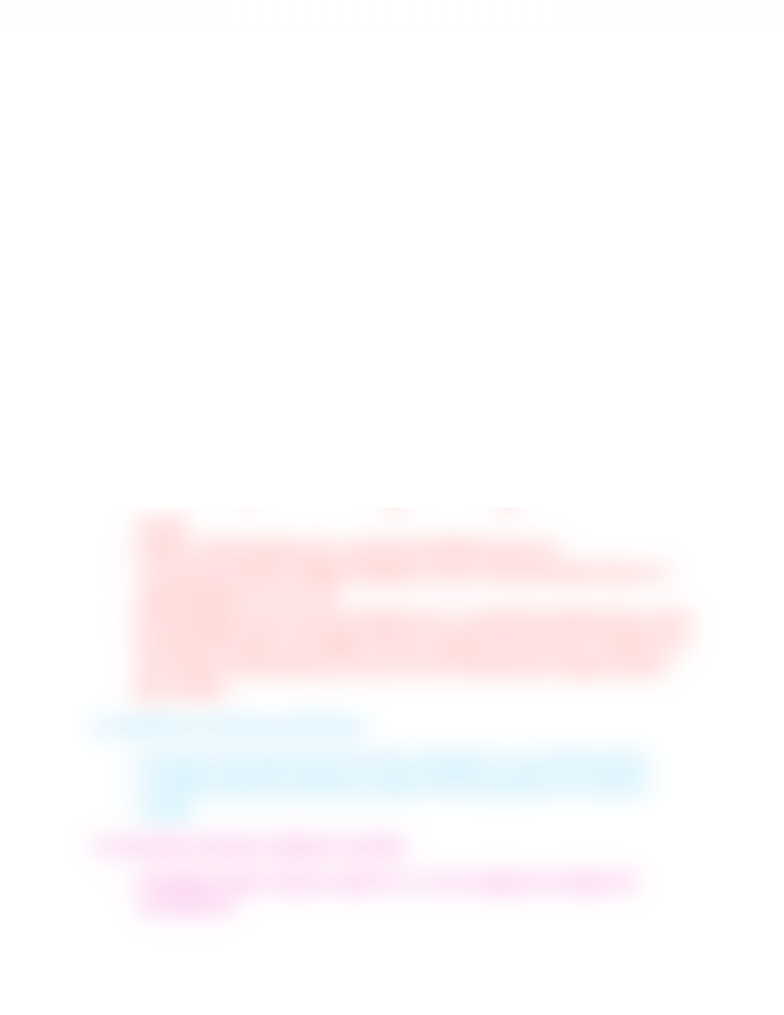# BIOL 2060 Lecture Notes - Mosaic Plot, Contingency Table, Scatter Plot

13 views2 pages30 Jan 2013
School
Department
Course
ProfessorDisplaying Data
2.1 Displaying frequency Distributions
- Relative Frequency: proportion of observations having a given measurement
(freq. /total number of observations)
- Relative freq. Distribution: shows the proportion of the occurrences of each value
in the data set
- Frequency table: text, showing the # of occur. In each category
- Bar graph : height of rectangular bars to visualize the frequency of each category
- You can organize the table by putting the most frequent at the top
- Rules for bar graph: baseline must be zero, and you must label the graph.
- Bars should be of the same width and start at the baseline and shouldn’t be fused
together, provide total number of observations in the figure legend.
- Histogram: uses area of rectangular bars to display frequency, splits into bins,
equal width, uses RANGES
- Mode (histogram): highest peak ..bimodal means 2 peaks
- Symmetric: two sides are mirror images
- Skewed: if not symmetric ...skewed right: tail on the right...skewed left: tail on
the left
- Outliers: extreme data points, removed if SHOWN to be errors
- To choose the width of ranges (histogram): look at if what the data shows on 2
separate graphs is true or not
- Rule (histogram): bars rise from baseline zero, no separation between bars, at the
boundary then place in the higher interval..sturges rule of thumb: 1+1(n)/ln2 (n is
the number of observations), but we use more intervals than sturges, break at
god numbers
2.2 Quantiles of a frequency distribution
- Percentile: value below which x% of the individuals lie...also X/100 quantile
- Cumulative frequency distribution: graph of all the quantiles of a numerical
variable
2.3 Associations between categorical variables
- Contingency table: frequency table for 2 or more categorical variables (all
combinations)
Unlock document

This preview shows half of the first page of the document.
Unlock all 2 pages and 3 million more documents.

# Get access

\$10 USD/m
Billed \$120 USD annually
Homework Help
Class Notes
Textbook Notes